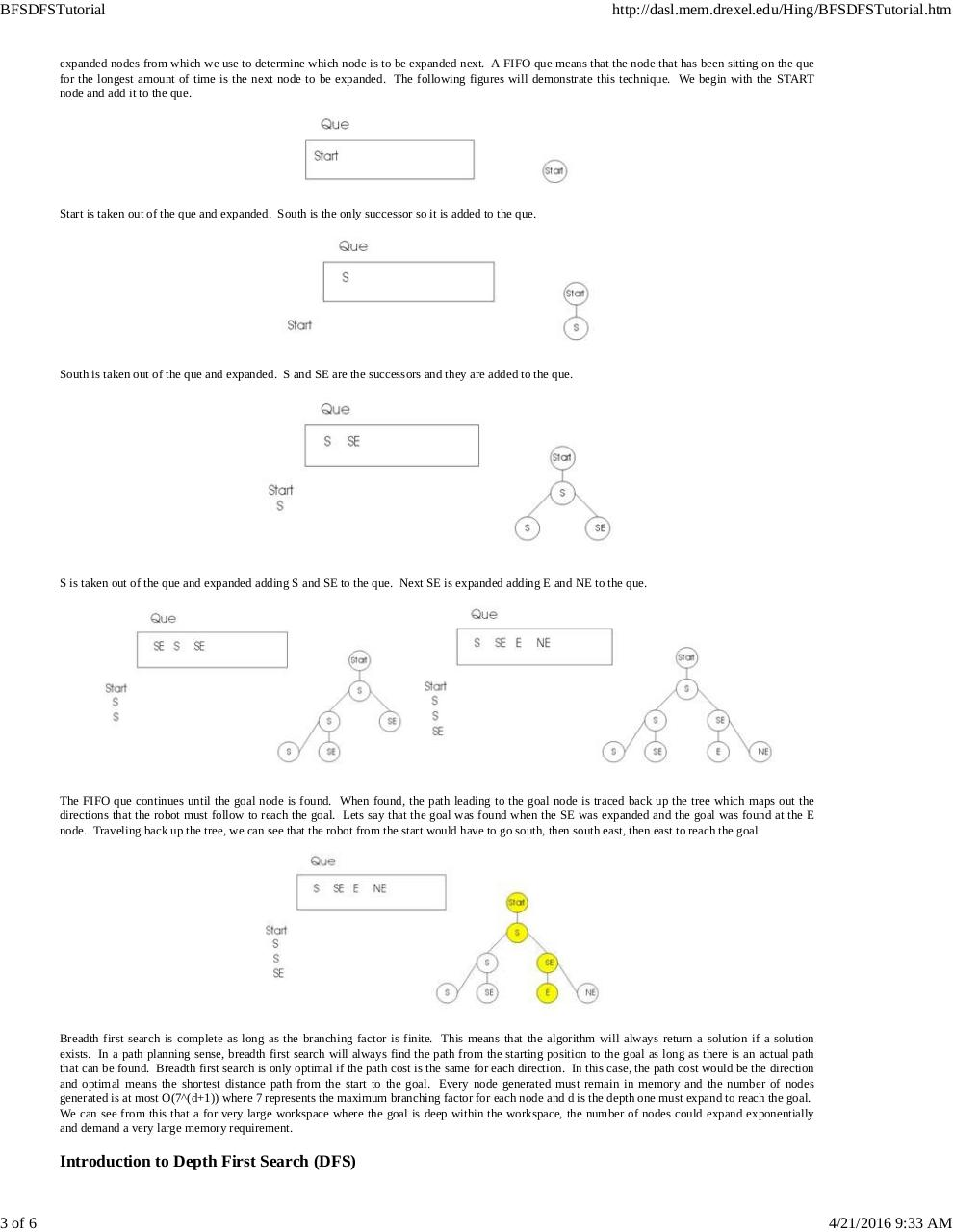# BFSDFSTutorial.pdfPage 1 2 3 4 5 6

#### Text preview

BFSDFSTutorial

3 of 6

http://dasl.mem.drexel.edu/Hing/BFSDFSTutorial.htm

expanded nodes from which we use to determine which node is to be expanded next. A FIFO que means that the node that has been sitting on the que
for the longest amount of time is the next node to be expanded. The following figures will demonstrate this technique. We begin with the START
node and add it to the que.

Start is taken out of the que and expanded. South is the only successor so it is added to the que.

South is taken out of the que and expanded. S and SE are the successors and they are added to the que.

S is taken out of the que and expanded adding S and SE to the que. Next SE is expanded adding E and NE to the que.

The FIFO que continues until the goal node is found. When found, the path leading to the goal node is traced back up the tree which maps out the
directions that the robot must follow to reach the goal. Lets say that the goal was found when the SE was expanded and the goal was found at the E
node. Traveling back up the tree, we can see that the robot from the start would have to go south, then south east, then east to reach the goal.

Breadth first search is complete as long as the branching factor is finite. This means that the algorithm will always return a solution if a solution
exists. In a path planning sense, breadth first search will always find the path from the starting position to the goal as long as there is an actual path
that can be found. Breadth first search is only optimal if the path cost is the same for each direction. In this case, the path cost would be the direction
and optimal means the shortest distance path from the start to the goal. Every node generated must remain in memory and the number of nodes
generated is at most O(7^(d+1)) where 7 represents the maximum branching factor for each node and d is the depth one must expand to reach the goal.
We can see from this that a for very large workspace where the goal is deep within the workspace, the number of nodes could expand exponentially
and demand a very large memory requirement.

Introduction to Depth First Search (DFS)

4/21/2016 9:33 AM